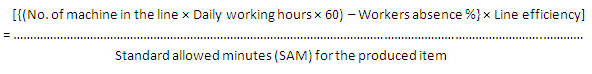# How to Calculate Sewing Line Capacity of an Apparel Industry?

### How to Calculate Sewing Line Capacity of an Apparel Industry?

Sewing line capacity is an important matter in the ready-made garments sector. By calculating sewing line capacity we can easily calculate the production capacity of any factory. As its importance, today I will present here the calculation method of sewing line’s capacity of the apparel industry.Sewing line capacity

#### The calculation method of sewing line capacity in the apparel industry:

To calculate the sewing lines capacity of a garment factory, an industrial engineer has needed the following information:

1. No. of sewing machines in the line,
2. Workers absence percentage of that line,
3. Daily working hours of that factory,
4. Line efficiency of that factory,
5. The standard allowed minutes (SAM) for the produced item.

Now, by using the below formula, an industrial engineer can easily calculate the sewing line’s capacity of the factory.

#### Sewing line’s capacity (In pcs),Example:

Suppose, in Abonty Fashions, a line is running for producing full sleeve shirt with 30 machines at 8hrs working day, where the line’s efficiency is 60% and worker absence percentage is 5%.

Find out the sewing lines capacity for the above factory.

#### Solution:

Here,

No. of sewing machines in line= 30,
Workers absence percentage of that line= 5%,
Daily working hours of that factory= 8,
Line efficiency of that factory= 60%,
The standard allowed minutes (SAM) for full sleeve shirt= 25.

So,

Sewing line capacity (In pcs),= 328.32

=328pcs

So, the sewing line’s capacity per day for the above factory is 328pcs.

Speech from the writer:

1. How to calculate the sewing line’s capacity of a garment factory?
2. How to calculate the sewing room capacity of a garment factory?
3. Describe the sewing section capacity calculation method for a garment factory.
4. Describe the sewing section capacity calculation method for the apparel industry.
5. Describe the sewing room capacity calculation method for the apparel industry.
6. Describe the sewing room capacity calculation method for a clothing factory.

### One comment

•A Velliangiri A

Comment tomorrow Thank you

This site uses Akismet to reduce spam. Learn how your comment data is processed.